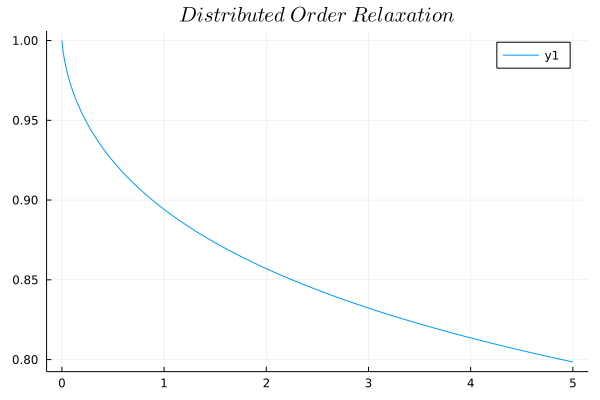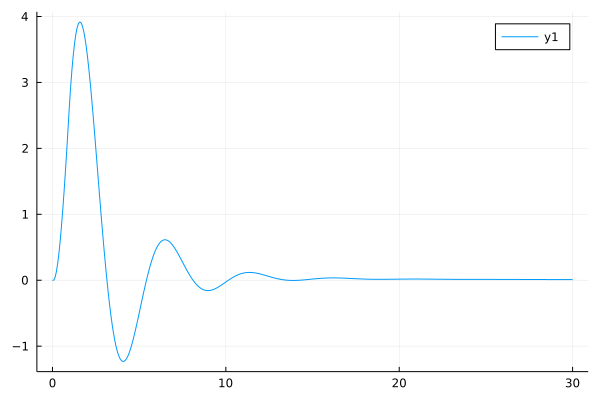# Distributed Order Differential Equations

For more details about distributed order diffeerntial equations and distributed order dynamic systems, we recommend you to read Distributed-Order Dynamic Systems

We integrate ${_0D_t^\alpha f(t)}$ with respect to the order, we can obtain distributed-order differential/integral equations. Since we normally adopted the notation as:

$$${_0D_t^{\omega(\alpha)} f(t)} := \int_{\gamma_1}^{\gamma_2}\omega(\alpha){_0D_t^\alpha f(t)}d\alpha$$$

We can write the general form of distributed order differential equations as:

$$$\int_0^m \mathscr{A}(r,\ D_*^r u(t))dr = f(t)$$$

Similar with what we have learned about single-term and multi-term fractional differential equations in linear fractional differential equations, we can also write the single-term distributed order differential equations:

$$$D_*^ru(t)=f(t,\ u(t))$$$

And multi-term distributed order differential equations

$$$\sum_{i=1}^k \gamma_i D_*^{r_i}u(t) = f(t,\ u(t))$$$

## Example1: Distributed order relaxation

The distributed order relaxation equation is similar with fractional relaxation equation, only the order is changed to a distributed function. Let's see an example here, the distributed order relaxation:

$$${_0D_t^{\omega(\alpha)} u(t)}+bu(t)=f(t),\quad x(0)=1$$$

With distribution of orders $\alpha$: $\omega(\alpha)=6\alpha(1-\alpha)$

By using the DOMatrixDiscrete method to solve this problem:

Info

The usage of DOMatrixDiscrete method is similiar with the FODEMatrixDiscrete method, all we need to do is to pass the parameters array and orders array to the problem difinition and solve the problem.

Tip

Pass the weight function and other orders to the order array is the right way:

julia> orders = [x->x*(1-x), 1.2, 3]
3-element Vector{Any}:
#3 (generic function with 1 method)
1.2
3
using FractionalDiffEq, Plots
h = 0.01; t = collect(h:h:5);
fun(t)=0
prob = DODEProblem([1, 0.1], [x->6*x*(1-x), 0], (0, 1), fun, 1, t)
sol = solve(prob, h, DOMatrixDiscrete())
plot(sol)## Example2: Distributed order oscillator

When the damping term in Bagley-Torvik equation described by a distributed-order derivative, we get the distributed order oscillator equation:

$$$ay''(t)+by^{\omega(\alpha)}(t)+cy(t)=f(t)$$$$$$f(t)=\begin{cases} 8, & (0\leq t\leq1)\\ 0, & (t>1) \end{cases}$$$
using FractionalDiffEq, Plots
h=0.075; t=collect(0:h:30)
f(t) = 0 ≤ t ≤ 1 ? (return 8) : (return 0)
prob=DODEProblem([1, 1, 1], [2, x->6*x*(1-x), 0], (0, 1), f, [0; 0], t)
sol=solve(prob, h, DOMatrixDiscrete())
plot(sol)Please see Distributed-Order Dynamic Systems for systematic introduction and knowledge.